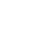Live chatA set is defined as a collection of infinite or finite objects in no clearly defined order (Weisstein, 2011, p. 1).

A set contains members which are referred to as elements of a set. Finite sets are characterized by countable number of elements. In an infinite set however it is impossible to list all the members of a set (Kuratowski and Mostowski, 1976, p 169).

Let set A be a list of five essential items which are; an introduction to statistics text book, a pair of shoes, a laptop, a leather bag and football sportswear.

## Buy Free Custom «The Quantitative Reasoning» Essay Paper paper online

* Final order price might be slightly different depending on the current exchange rate of chosen payment system.

Let set B which is a set of the fun stuffs contain the items; a laptop, an ipod, a skipping rope, a skate board and football sportswear.

The prices of the introduction to statistics textbook, the leather bag, the pair of shoes, the laptop, and the football sportswear are 80, 70, 20, 60 and 40 respectively. This will be represented by set A where the elements of the set will illustrate the prices of the items.

Set A is given by A= {20, 40, 60, 70, 80}

The prices of the ipod, skipping rope, skateboard, laptop and the football sportswear are 120, 30, 90, 60 and 40 respectively. The prices of these non-essential items are denoted by set B.

The identical items in both sets are the laptop and the football sportswear.

While set B is described by B= {30, 40, 60, 90, 120}

The union of a set is defined as the set of elements which are in set A or in set B or in both sets.

The union of set A and set B is given by

A %u1D17 B = {20, 30, 40, 60, 70, 80, 90, 120}

The intersection of a set is described as the set which contains the elements common to both set A and set B.

Stay ConnectedThe intersection of set A and set B is given by

A %u1D16 B = {40, 60}

The total cost of set A when it contains a unit of each item is given by:

Set is given by A= {20, 40, 60, 70, 80}

Therefore the total cost is {20+40+60+70+80} = 270

The union of set A and set B is given by:

A %u1D17 B = {20, 30, 40, 60, 70, 80, 90, 120}

The total cost of the union of set A and set B

Total cost is {20+ 30+40+ 60+ 70+ 80 +90+ 120} = 510

The intersection is given by:

A %u1D16 B = {40, 60}

Thus the total cost of the intersection is {40+60} = 100

The union of two set A’s will be set A since the union is the list of all the items in both sets with no elements listed more than once (onlinemathlearning.com, n.d p1).

Limited time Offer

0
0
days
:
0
0
hours
:
0
0
minutes
:
0
0
seconds
Get 19% OFF

A= {20, 40, 60, 70, 80} and set B= {30, 40, 60, 90, 120}

Thus the union of three set B’s and two set A’s is given by:

A %u1D17 B = {20, 30, 40, 60, 70, 80, 90, 120}

And the intersection is given by:

A %u1D16 B = {40, 60}

Therefore, as calculated earlier, the total cost of the union and intersection of three set B’s and two set A’s is given 510 and 100 respectively.

#### Related Accounting essays

0

Preparing Orders

0

Active Writers

0

Support Agents

Limited offerget 15% off your 1st order